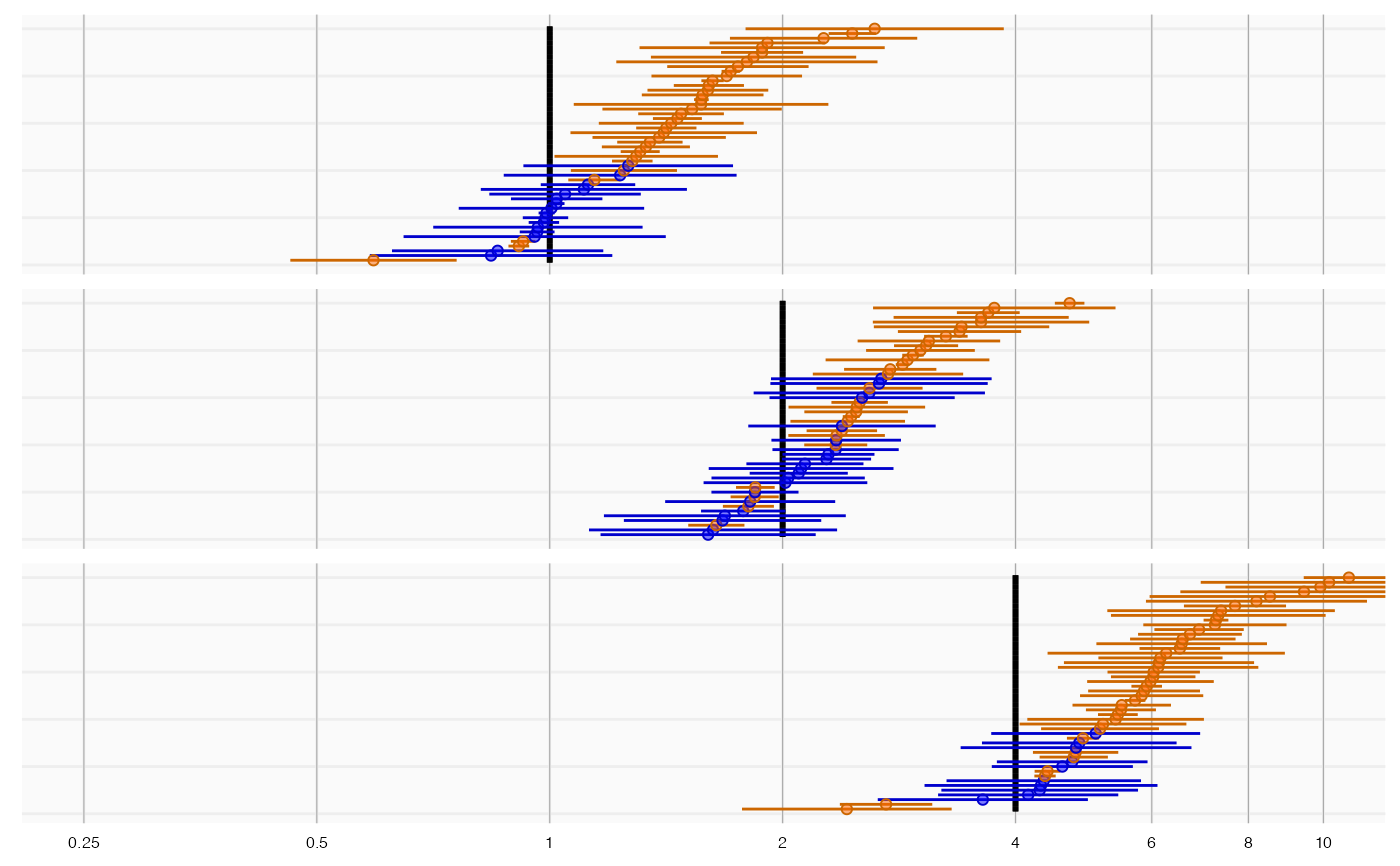Simulate (negative) controls

simulateControls(
n = 50,
mean = 0,
sd = 0.1,
seLogRr = runif(n, min = 0.01, max = 0.2),
trueLogRr = 0
)

## Arguments

n

Number of controls to simulate.

mean

The mean of the error distribution (on the log RR scale).

sd

The standard deviation of the error distribution (on the log RR scale).

seLogRr

The standard error of the log of the relative risk. This is recycled for the controls. The default is to sample these from a uniform distribution.

trueLogRr

The true relative risk (on the log scale) used to generate these controls. This is recycled for the controls.

## Details

Generate point estimates given known true effect sizes and standard errors

## Examples

data <- simulateControls(n = 50 * 3, mean = 0.25, sd = 0.25, trueLogRr = log(c(1, 2, 4)))
plotTrueAndObserved(data$logRr, data$seLogRr, data\$trueLogRr)